$\newcommand{\dotp}{\langle #1, #2 \rangle}$ $\newcommand{\enscond}{\lbrace #1, #2 \rbrace}$ $\newcommand{\pd}{ \frac{ \partial #1}{\partial #2} }$ $\newcommand{\umin}{\underset{#1}{\min}\;}$ $\newcommand{\umax}{\underset{#1}{\max}\;}$ $\newcommand{\umin}{\underset{#1}{\min}\;}$ $\newcommand{\uargmin}{\underset{#1}{argmin}\;}$ $\newcommand{\norm}{\|#1\|}$ $\newcommand{\abs}{\left|#1\right|}$ $\newcommand{\choice}{ \left\{ \begin{array}{l} #1 \end{array} \right. }$ $\newcommand{\pa}{\left(#1\right)}$ $\newcommand{\diag}{{diag}\left( #1 \right)}$ $\newcommand{\qandq}{\quad\text{and}\quad}$ $\newcommand{\qwhereq}{\quad\text{where}\quad}$ $\newcommand{\qifq}{ \quad \text{if} \quad }$ $\newcommand{\qarrq}{ \quad \Longrightarrow \quad }$ $\newcommand{\ZZ}{\mathbb{Z}}$ $\newcommand{\CC}{\mathbb{C}}$ $\newcommand{\RR}{\mathbb{R}}$ $\newcommand{\EE}{\mathbb{E}}$ $\newcommand{\Zz}{\mathcal{Z}}$ $\newcommand{\Ww}{\mathcal{W}}$ $\newcommand{\Vv}{\mathcal{V}}$ $\newcommand{\Nn}{\mathcal{N}}$ $\newcommand{\NN}{\mathcal{N}}$ $\newcommand{\Hh}{\mathcal{H}}$ $\newcommand{\Bb}{\mathcal{B}}$ $\newcommand{\Ee}{\mathcal{E}}$ $\newcommand{\Cc}{\mathcal{C}}$ $\newcommand{\Gg}{\mathcal{G}}$ $\newcommand{\Ss}{\mathcal{S}}$ $\newcommand{\Pp}{\mathcal{P}}$ $\newcommand{\Ff}{\mathcal{F}}$ $\newcommand{\Xx}{\mathcal{X}}$ $\newcommand{\Mm}{\mathcal{M}}$ $\newcommand{\Ii}{\mathcal{I}}$ $\newcommand{\Dd}{\mathcal{D}}$ $\newcommand{\Ll}{\mathcal{L}}$ $\newcommand{\Tt}{\mathcal{T}}$ $\newcommand{\si}{\sigma}$ $\newcommand{\al}{\alpha}$ $\newcommand{\la}{\lambda}$ $\newcommand{\ga}{\gamma}$ $\newcommand{\Ga}{\Gamma}$ $\newcommand{\La}{\Lambda}$ $\newcommand{\si}{\sigma}$ $\newcommand{\Si}{\Sigma}$ $\newcommand{\be}{\beta}$ $\newcommand{\de}{\delta}$ $\newcommand{\De}{\Delta}$ $\newcommand{\phi}{\varphi}$ $\newcommand{\th}{\theta}$ $\newcommand{\om}{\omega}$ $\newcommand{\Om}{\Omega}$

This tour explores the resolution of the shape from shading inverse problem using Fast Marching.

In :
addpath('toolbox_signal')


## Forward Image Formation¶

We consider here a simplified imaging system where the camera performs an orthogonal projection and the lighting is orthogonal to the camera focal plane and is at infinity. We also assume that the surface is perfectly diffuse (Lambertian).

Shape From Shading, Emmanuel Prados, Olivier Faugeras Handbook of Mathematical Models in Computer Vision, Springer, page 375-388, 2006.

The shape use in this tour is taken from

Shape from Shading: A Survey, Ruo Zhang, Ping-Sing Tsai, James Edwin, Cryer and Mubarak Shah, IEEE Trans. on PAMI, Vol. 21, No. 8, 1999.

Load a surface as a 2D height field, i.e. it is defined using an image $f(x,y)$.

In :
name = 'mozart';
n = size(f,1);


Height $h>0$ of the surface.

In :
h = n*.4;


Final rescale surface $(x,y,f(x,y)) \in \RR^3$.

In :
f = rescale(f,0,h);


Display the image.

In :
clf;
imageplot(f);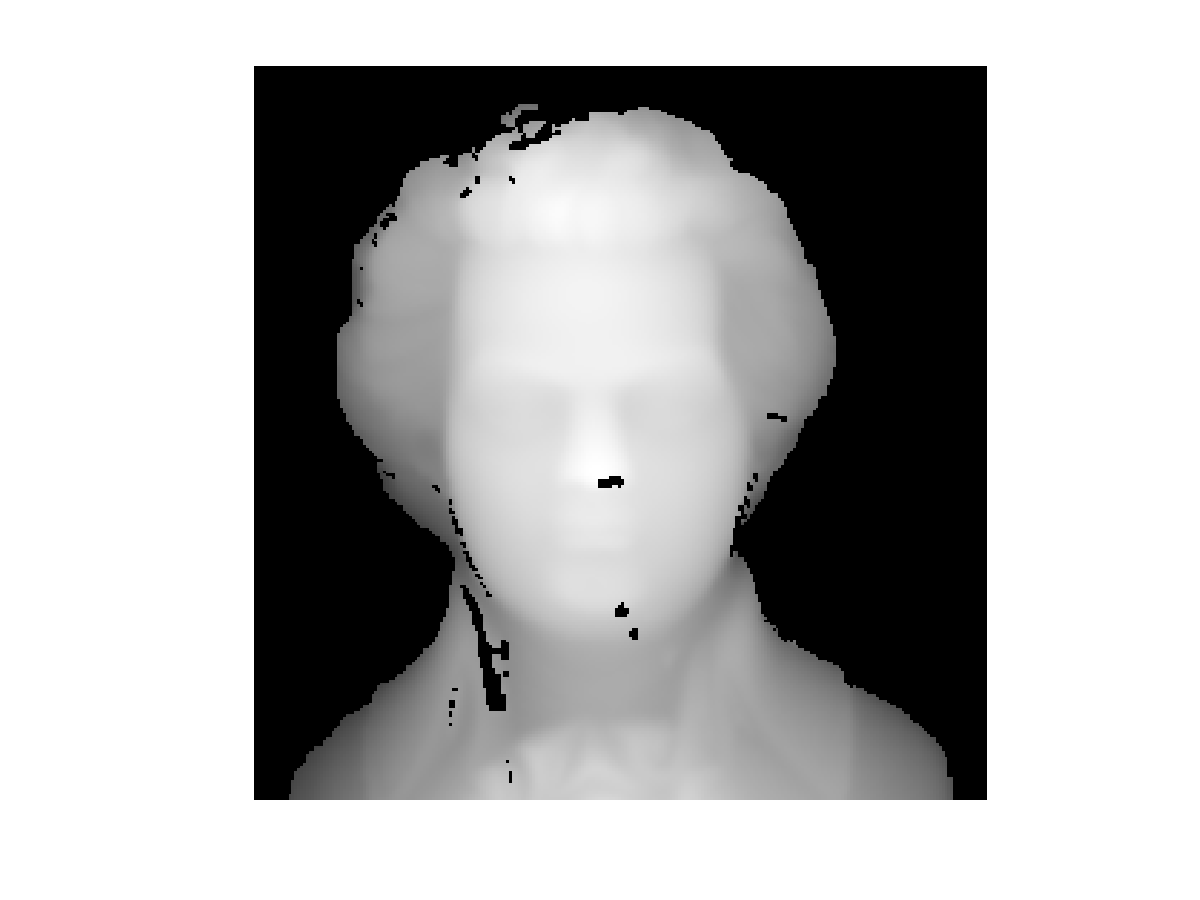Display as a 3-D surface.

In :
clf;
surf(f);
colormap(gray(256));
axis equal;
view(110,45);
axis('off');
camlight;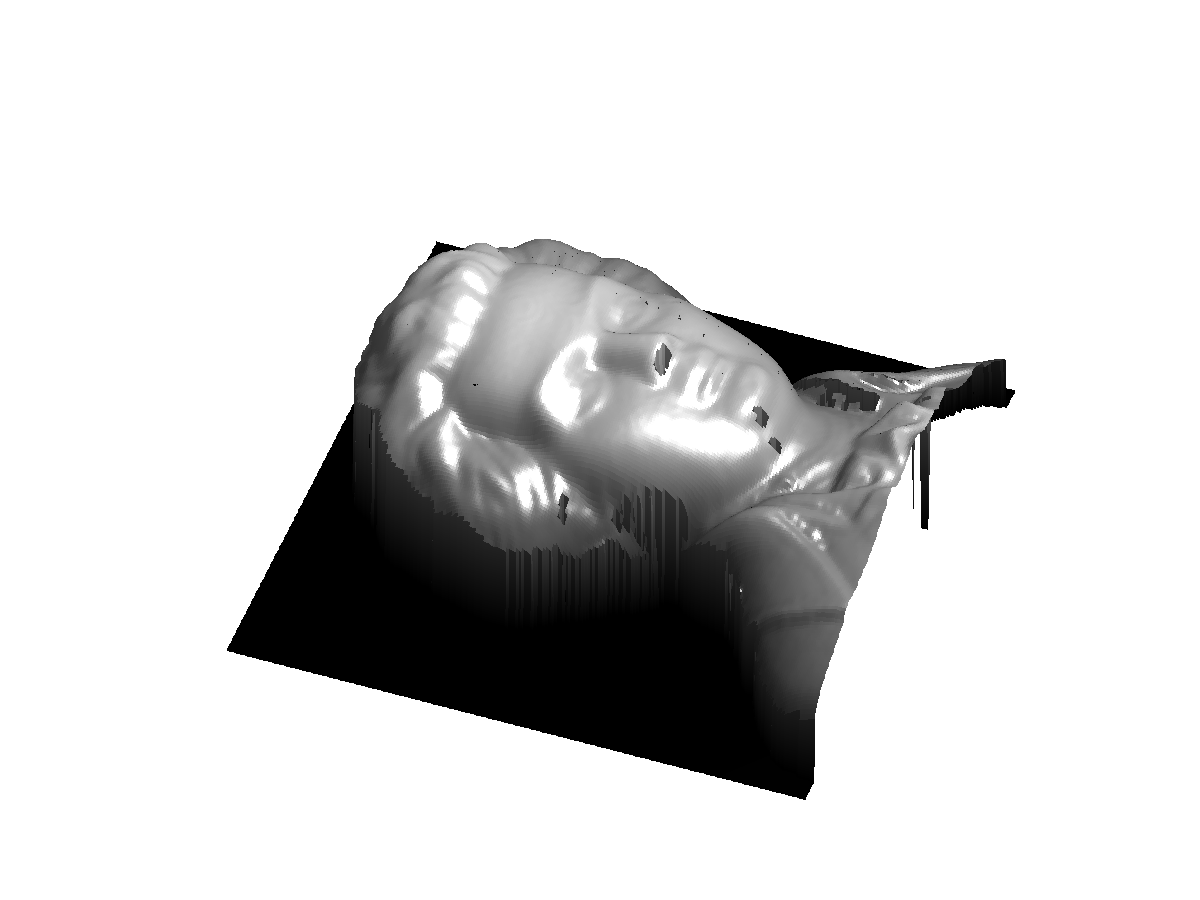Compute the normal to the surface $(x,y,f(x,y))$. The tangent vectors are $(1,0,\pd{f}{x}(x,y))$ and $(0,1,\pd{f}{y}(x,y))$ and the normal is thus $$N(x,y) = \frac{N_0(x,y)}{\norm{N_0(x,y)}} \in \RR^3 \qwhereq N_0(x,y) = \pa{\pd{f}{x}(x,y), \pd{f}{y}(x,y), 1}.$$

Compute $N_0$, the un-normalized normal, using centered finite differences.

In :
options.order = 2;


Compute the normalized normal $N$.

In :
s = sqrt( sum(N0.^2,3) );
N = N0 ./ repmat( s, [1 1 3] );


Display the normal map as a color image.

In :
clf;
imageplot(N);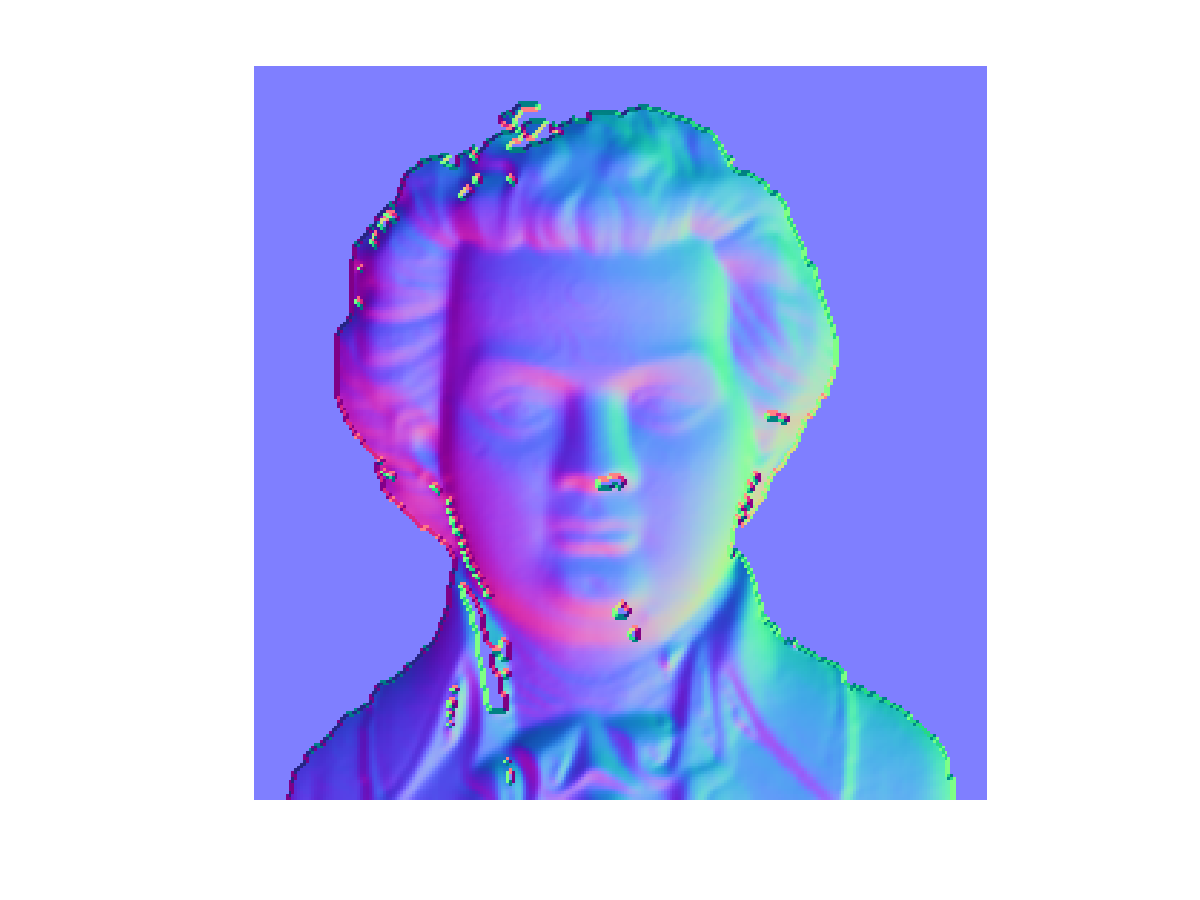We compute the shaded image obtained by an infinite light coming from a given direction $d \in \RR^3$.

In :
d = [0 0 1];


We use a Labertian reflectance model to determine the luminance $$L(x,y) = (\dotp{N(x,y)}{ d })_+ \qwhereq (\al)_+ = \max(\al,0).$$

In :
L = max(0, sum( N .* repmat(reshape(d,[1 1 3]), [n n 1]),3 ) );


Display the lit surface.

In :
vmin = .3;
clf;
imageplot(max(L,vmin));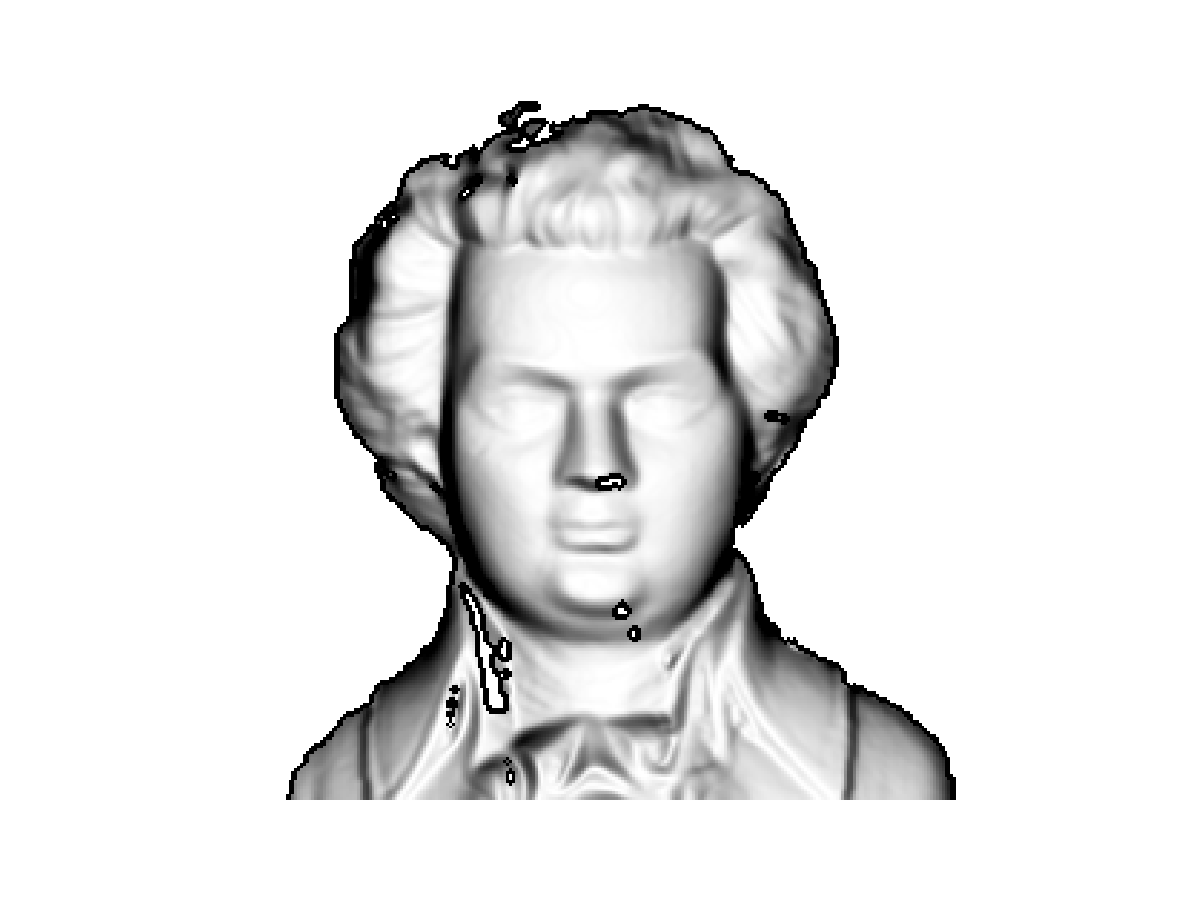Exercise 1

Display the surface with several light directions $d \in \RR^3$.

In :
exo1()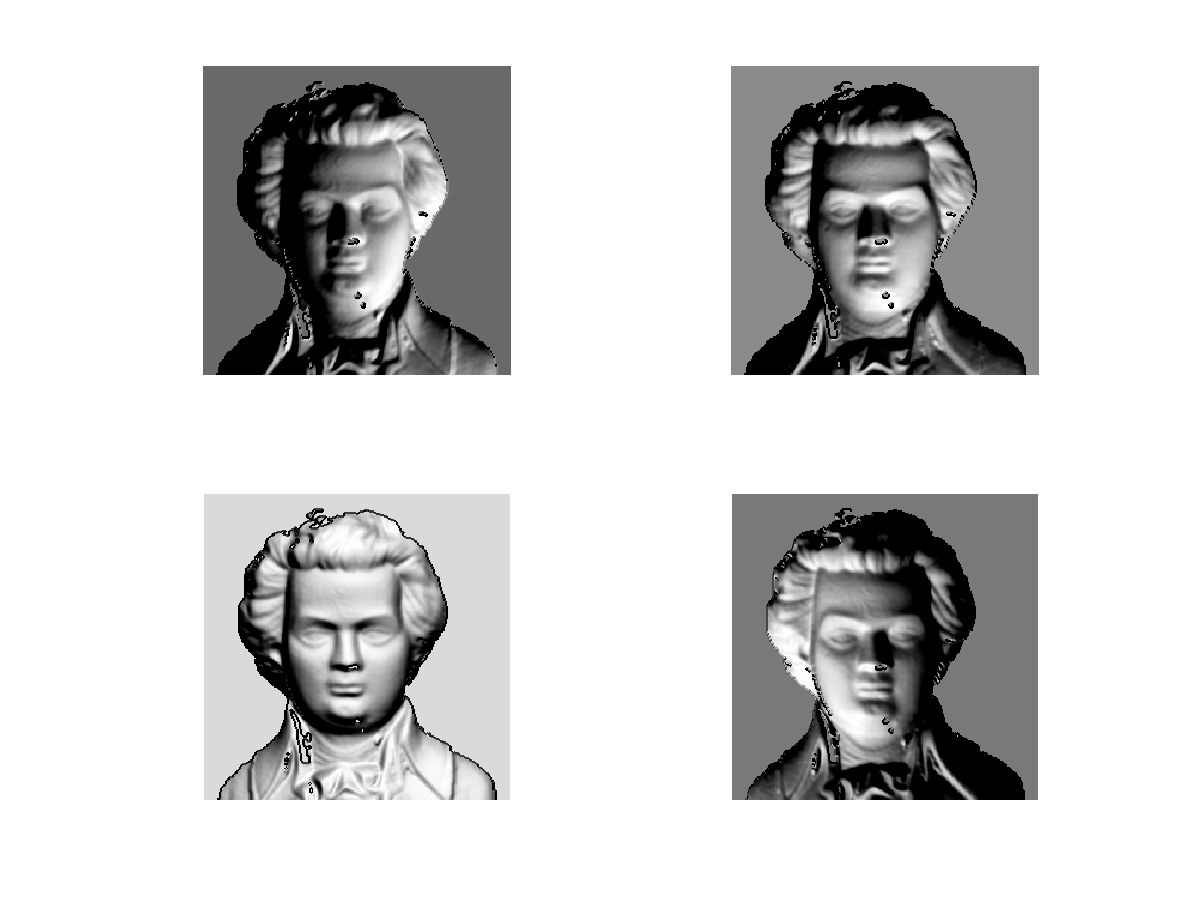In :
%% Insert your code here.


For a vertical ligthing direction $d=(0,0,1)$, the forward imaging operator reads $$L(x,y) = \frac{1}{ \sqrt{ \norm{ \nabla f(x,y) }^2+1 } }.$$

Compute the luminance map for $d=(0,0,1)$.

In :
d = [0 0 1];
L = sum( N .* repmat(reshape(d,[1 1 3]), [n n 1]),3 );


The height field $f$ can thus, in theory, be recovered by solving the following Eikonal equation: $$\norm{ \nabla f(x,y) } = W(x,y) \qwhereq W(x,y) = \sqrt{ 1/L(x,y)^2 - 1 }$$ With additional boundary conditon $$\forall i \in I, \quad f( p_i) = f_i$$ For a set of well chosen grid points $p_i \in \RR^2$.

The issue is that this equation is ill-posed (several solution) due to the singular points $(q_j)_{j \in J}$ where $L(q_j)$ is close to 1, so that $W(q_j)$ is close to 0. A way to regularize the inversion is thus to choose the boundary condition points $p_i$ to be the singular points $q_j$.

Set a tolerance $\epsilon>0$.

In :
epsilon = 1e-9;


We show in white the location of singular points $q_j$, that satisfies $L(q_j)>1-\epsilon$.

In :
clf;
imageplot(L>1-epsilon);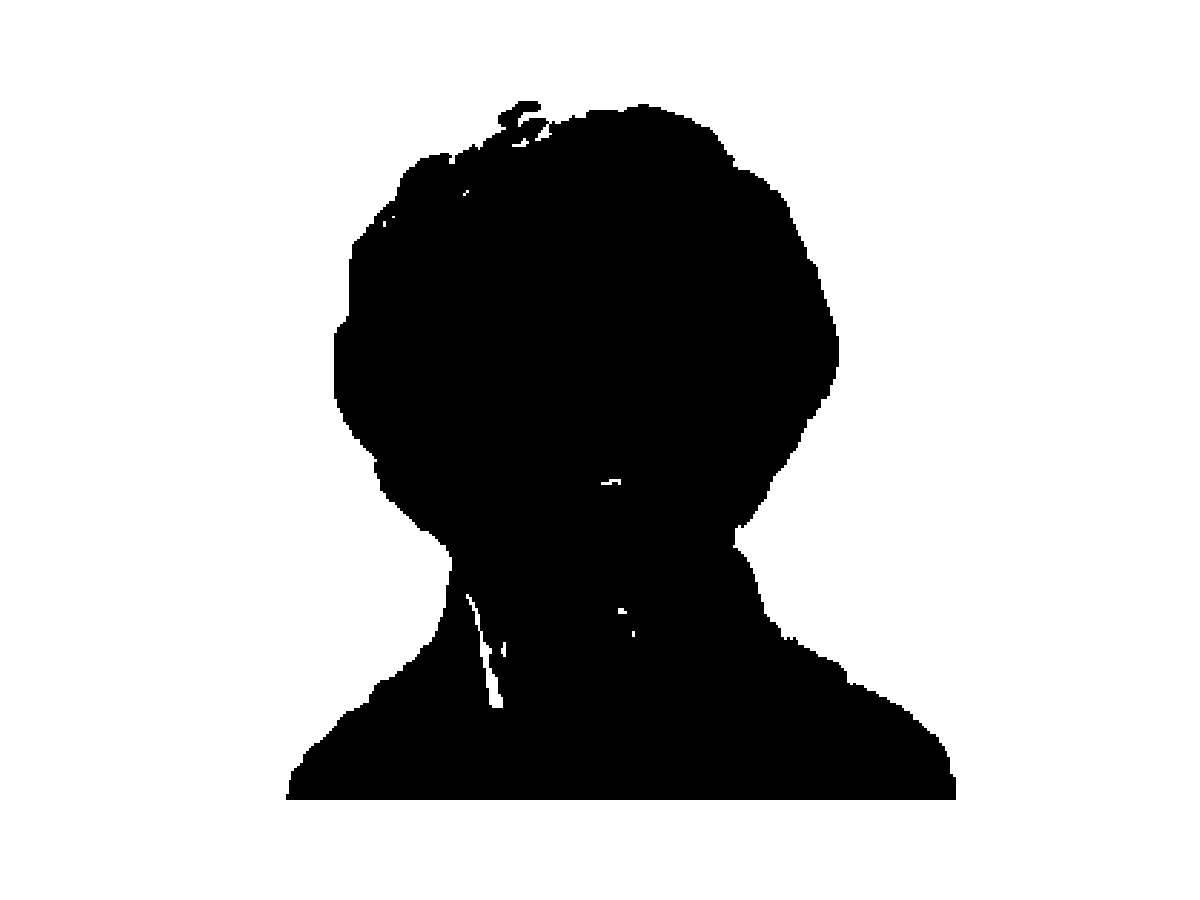Compute the Eikonal speed $W$ (right hand side of the equation).

In :
W = sqrt(1./L.^2-1);


To avoid too much ill-posedness, we threshold it.

In :
W = max(W,epsilon);


Display the speed.

In :
clf;
imageplot(min(W,3));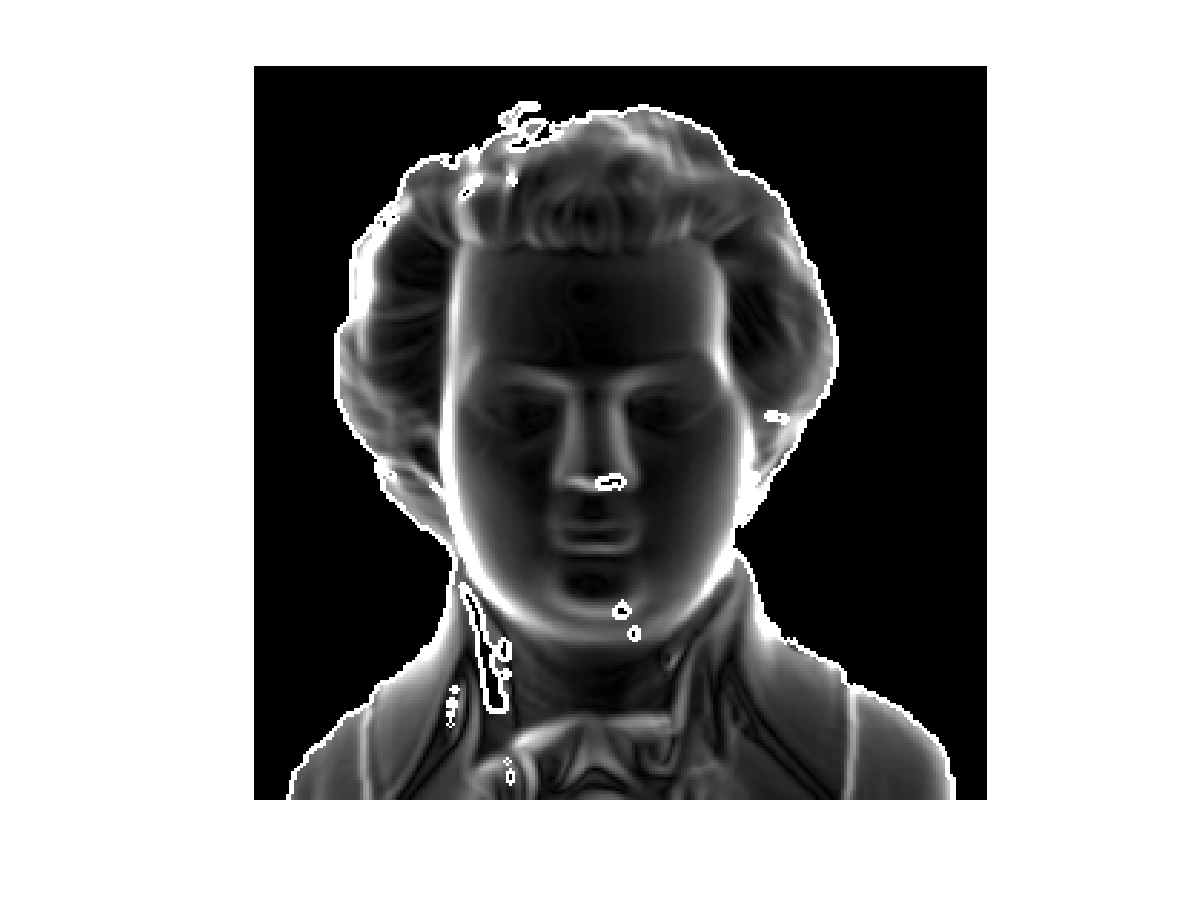We use here a single point $p$. Select the tip of the nose as base point $p$.

In :
p = [140;120];


Solve the Eikonal equation, assuming $f(p)=0$.

In :
[f1,S] = perform_fast_marching(1./W, p);


Rescale the height.

In :
f1 = -f1*n;


Display as a 3D surface.

In :
clf;
hold on;
surf(f1);
h = plot3(p(2), p(1), f1(p(1),p(2)), 'r.');
set(h, 'MarkerSize', 30);
colormap(gray(256));
axis('equal');
view(110,45);
axis('off');
camlight;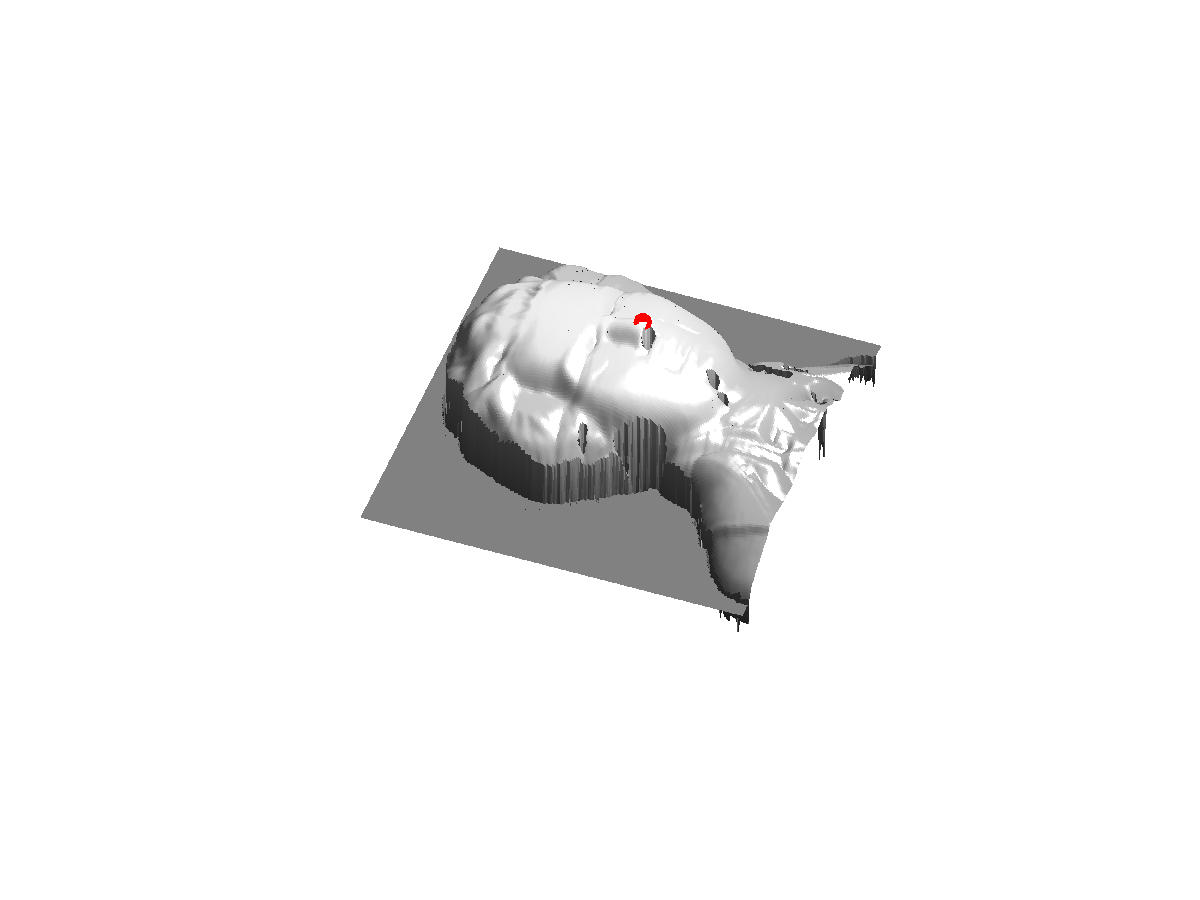Exercise 2

Try to reconstruct the image starting from other base points. What do you observe ?

In :
exo2()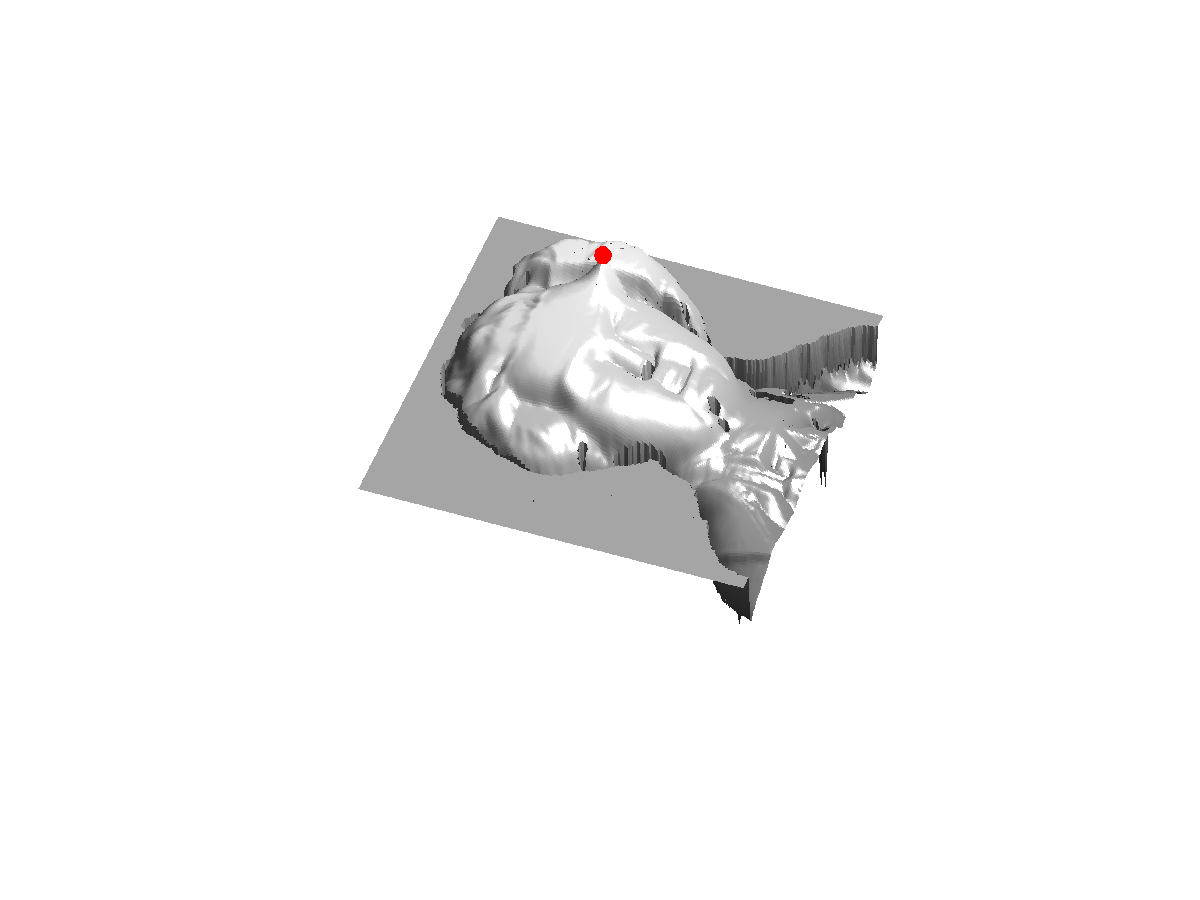In :
%% Insert your code here.


Exercise 3

Try to improve the quality of the reconstruction by selecting several points, and imposing their height.

In :
exo3()

In :
%% Insert your code here.